# Questions to do with crankshaft of an engine

## Homework Statement

A cylinder in a truck engine are shown in
Figure Q8.1 below. The piston slides without friction in the cylinder of diameter
150 mm. The combustion chamber is subjected to an internal pressure of
50 bar gauge as a result of fuel combustion without any leakage past the piston.
The connecting rod AB has a length of 200 mm. It is linked to the piston at A
and to the crank-shaft at B. The crank-shaft OB has a revolution radius of
75 mm

1 - Calculate the resulting instantaneous force on the piston if downward force is 80kN about the crankshaft axis of rotation O..
2 - Calculate the angle OAB.
3 - Calculate the projection of the force transmitted by the piston to connecting rod AB.
4 - Isolate crank pin OB and calculate the moment resulting from the force transmitted by connecting rod AB.
Consider an anti-clockwise rotation as positive.
5 - Calculate the rotational velocity of the engine crankshaft if engine is running at 1500rpm.
6 - What is the crankshaft power output of a 6 cylinder engine at this running
condition if the average torque produced on the crankshaft at a certain
engine load in one cylinder at 1500 rpm is 170 Nm?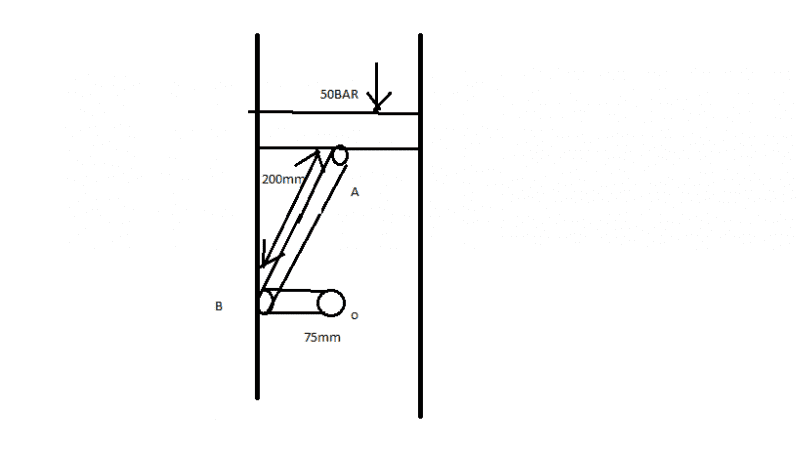## The Attempt at a Solution

1 - 50BAR = 5,000,000Pa, P=F/A so F=PA.
A = pi r^2, r= 0.075 so area = 0.01767m^2.
F=5,000,000 x 0.01767 = 88350N

2 - Length AB = 0.2m OB= 0.075m
therefore OAB= sin (0.075/0.2) = 22.02 degrees.

3 - Projection of force, 80,000 = adjacent, therefore Force on AB = 80,000/cos(22) = 86,282.8N

4 - Moment on OB from AB = 80,000 x 0.075 = 6000Nm as only vertical force of 80,000N can act on OB?

5 - Rotational velocity = 2pi x f.
1500rpm = 25Hz, therefore Rotational velocity = 2pi x 25 = 157.08 rad/s

6 - Torque = 170Nm, Power = Work/time
Work = 170 x 1500 x 6 = 1,530,000N for all 6 cylinders per minute
Power = 1,530,000/ 60 seconds = 25,500W

Thank you, I hope these are mostly right. I'm not sure about last one.

Last edited by a moderator:

CWatters
Homework Helper
Gold Member
Your answer for 1) seems ok but appears to ignore the bit I've underlined below.

1 - Calculate the resulting instantaneous force on the piston if downward force is 80kN about the crankshaft axis of rotation O.

What does that bit mean anyway? What exactly is a "downward force about a crankshaft axis of rotation" ? Is that the exact wording?

Hi,
This was the exact working from the test:
"Calculate the instantaneous torque produced by a downwards piston force
of 80 kN about the crankshaft axis of rotation O at the position shown in
Figure Q8.1"
I have no idea, it seemed confusing

CWatters
Homework Helper
Gold Member
1 - Calculate the resulting instantaneous force on the piston if downward force is 80kN about the crankshaft axis of rotation O.

Calculate the instantaneous torque produced by a downwards piston force of 80 kN about the crankshaft axis of rotation O at the position shown in Figure Q8.1"

:)

So your answer to Q1 is incorrect. They aren't asking you to calculate the force on the piston. They are telling you it's about 80KN and are asking you to calculate the torque on the crankshaft.

We have a webpage where we do the test, and it says calculate the FORCE, but it also gives us a test paper and refers us to the question to answer which gives us the details of the situation. In the test paper it says torque not force, so I'm not sure which one we are supposed to do

NascentOxygen
Staff Emeritus
Perhaps clearer:

Calculate the instantaneous torque about the crankshaft axis of rotation O (at the position shown in
Figure Q8.1) which would be produced by a downwards piston force of 80kN.

http://thumbnails112.imagebam.com/37333/0363e9373324851.jpg [Broken]

Last edited by a moderator:
So was my method correct?

CWatters
Homework Helper
Gold Member
We have a webpage where we do the test, and it says calculate the FORCE, but it also gives us a test paper and refers us to the question to answer which gives us the details of the situation. In the test paper it says torque not force, so I'm not sure which one we are supposed to do

I think you will have to ask for clarification. If that's not possible I would answer as you have done with the force = 88,350N.

Questions 2, 3 and 4 appear to rely on the answer to question 1 being a force. They lead on naturally from one to the other.

If the answer to Question 1 was the torque then you would need to work out the answers to questions 2,3 and 4 first in order to solve Question 1.

I just sent the lecturer an email asking to clarify. Questions 2, 3 and 4 say assume it is 80,000N though?

The first question is:
Calculate the resulting force on the piston from the pressure in the
combustion chamber.
So was what I did for this and the following question right?

So on the first one you do not need to assume downwards force is 80kN, but on the second question onwards you do.

So if it's from the pressure I believe I have done it correctly.
However, question 2 says to find the angle OAB from the question in the test paper: "
Calculate the instantaneous torque produced by a downwards piston force
of 80 kN about the crankshaft axis of rotation O at the position shown in
Figure Q8.1." So I am unsure if I did this correctly, as force doesn't seem to affect the angle it is the length of the crankshaft that dictates the angle?
thanks.

CWatters
Homework Helper
Gold Member
Q2 - Calculate the angle OAB.
A2 - Length AB = 0.2m OB= 0.075m
therefore OAB= sin (0.075/0.2) = 22.02 degrees.

OAB = 22 degrees is correct.

Q3 - Calculate the projection of the force transmitted by the piston to connecting rod AB.
A3 - Force on AB = 80,000/cos(22) = 86,282.8N

I don't think that's correct. If the force from the piston is FP acting vertically then the force acting in the direction of the crank is

FpCos(22)

Q4 - Isolate crank pin OB and calculate the moment resulting from the force transmitted by connecting rod AB.
A4 - Moment on OB from AB = 80,000 x 0.075 = 6000Nm as only vertical force of 80,000N can act on OB?

Not sure about that. I would use the force in the direction of the connecting rod (eg FPCos(22)) and this diagram to calculate the tangential distance...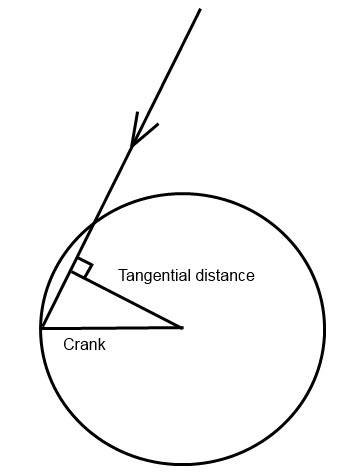Thank you for the help! Why is it cos(22)? isn't the force downwards the adjacent, because it looked like the connecting rod AB would be the hypotenuse?
Q4 - I don't quite understand what it is I am supposed to do for this one, which force component am I finding exactly?
Thanks

Last edited:
Actually, for Q4. I could find the angle ABO, then use the force of the connecting rod Fp cos (22) to find the adjacent of the triangle in the diagram?

Just looked at the question 4 again it says: Calculate the projection of the force transmitted by the piston to connecting rod AB.
So you are finding the force on AB not the crank. Is the 80kN force by the piston not going to be acting as the adjacent as the angle is 22 degrees between the 80kN and AB? so connecting rod AB is going to be the hypotenuse?

CWatters
Homework Helper
Gold Member

I answered that above when I wrote this earlier... "I think you will have to ask for clarification. If that's not possible I would answer as you have done with the force = 88,350N."

Thank you for the help! Why is it cos(22)? isn't the force downwards the adjacent, because it looked like the connecting rod AB would be the hypotenuse?

When you resolve a force (eg FP) into two components the components need to be at right angles or they aren't independent of each other. (If they aren't orthogonal then one component would itself have a component in the direction of the other.)

In this problem we want one of the components to be in the direction of the con rod so the other will be at right angles to the con rod. If you draw that you get this..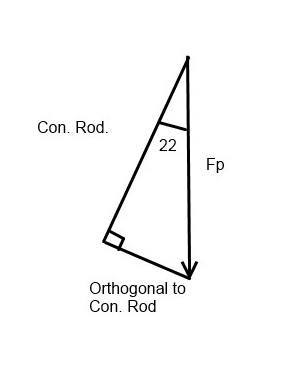Cos(22) = Fconrod/Fp
or
Fconrod = FpCos(22)

Last edited:
CWatters
Homework Helper
Gold Member
I'm getting really confused with the question numbers. In the original post Q4 says...

4 - Isolate crank pin OB and calculate the moment resulting from the force transmitted by connecting rod AB.
Consider an anti-clockwise rotation as positive.

That's asking you to calculate the moment/torque that the con rod gives the crankshaft. For that see the diagram in post #12.

Torque = Fconrod * tangential distance

From above

Fconrod = FpCos(22)

From the diagram in #12..

Tangential distance = LcrankCos(22)

So

Torque = FpCos(22) * LcrankCos(22)
=Fp*LcrankCos(22)2

Fp is either 88,350N (if you calculate it from the pressure) or 80,000N (if you use the value the question tells you to assume). Hopefully your tutor will clarify that issue.

I know it is very confusing for me too, I'm sorry at the mess of this. It's stupid how they've done this test with the different set of questions it has made it very complicated.

The first question said to find the resultant force from the pressure, so I'm pretty sure I've done that right.
Then for question involving the diagram you just sent, why is it a right angle between the connecting rod and crank shaft? because it makes sense how that is the hypotenuse in that case but in the diagram it is perpendicular to the cylinder walls.

I'm really sorry about the confusion, I'm so confused you must be getting annoyed with me!

I'm gonna try and start fresh:
The questions we are having troubles with:
1:
Calculate the projection of the force transmitted by the piston to connecting rod AB.
2:
Isolate crank pin OB and calculate the moment resulting from the force transmitted by connecting rod AB.
Consider an anti-clockwise rotation as positive.

Both these questions are linked to a single question from the test paper link we have been given which says "
Calculate the instantaneous torque produced by a downwards piston force
of 80 kN about the crankshaft axis of rotation O at the position shown in
Figure Q8.1." So we have to answer the original question from this question.

I hope this clears things up?
Thank you for putting up with this!

CWatters
Homework Helper
Gold Member
Then for question involving the diagram you just sent, why is it a right angle between the connecting rod and crank shaft? because it makes sense how that is the hypotenuse in that case but in the diagram it is perpendicular to the cylinder walls.

If you mean this diagram..It's not a right angle between the connecting rod and crank. The crank is horizontal in my diagram. Take a look at how torque is calculated. Only the tangential component of the force contributes to torque.

There are two ways to work that out but both give the same answer. In the first you work out the effective length of the crank (the tangential distance) in the second you work out the component of the force that is tangential to the crank. Both methods give the same answer... but perhaps the second is easier to understand?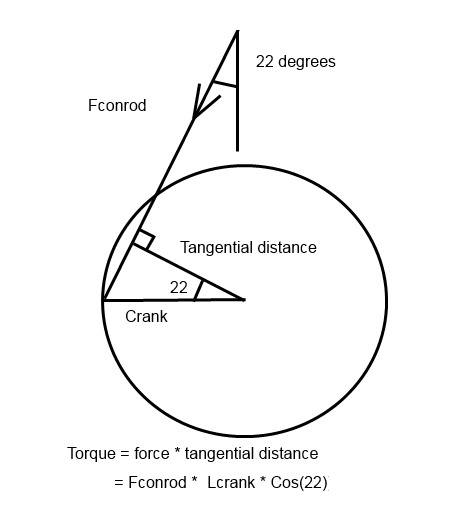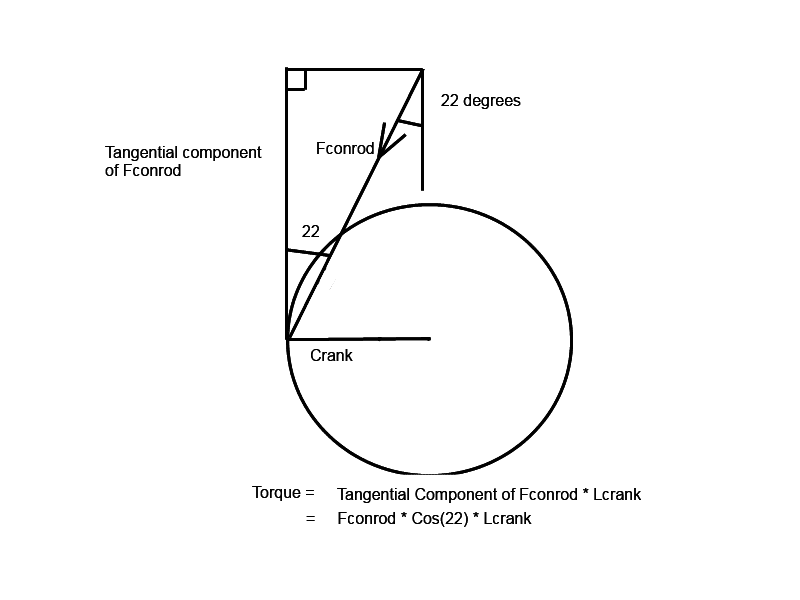CWatters
Homework Helper
Gold Member
So step by step starting from the top of the engine...

Vertical force from piston is..

Fp = 80kN (we are told it's 80kN but it calculates to 88,350 N)

The force in the conrod is..

Fconrod = Fp * Cos(22)

The torque on the crank is..

Tcrank = Fconrod * Cos(22) * Lcrank

Substituting you get..

Tcrank = Fp * Lcrank * Cos(22)2

Edit|; I make it 5158NM if Fp = 80,000N or 5696NM if Fp = 88,350N

Thank you this has helped me so much I understand it now, the only thing I am have trouble with is why is the last bit Cos(22)^2?

How are the last two questions? Then they'd be all done! I think the rotational velocity one is right, not certain about the last one.
Is there a way to add rep on this forum? I need to give you a whole bunch! :)

Oh wait I see how you got the cosine squared now!

CWatters
Homework Helper
Gold Member
5 - Calculate the rotational velocity of the engine crankshaft if engine is running at 1500rpm.

5 - Rotational velocity = 2pi x f.
1500rpm = 25Hz, therefore Rotational velocity = 2pi x 25 = 157.08 rad/s

That's correct.

6 - What is the crankshaft power output of a 6 cylinder engine at this running
condition if the average torque produced on the crankshaft at a certain
engine load in one cylinder at 1500 rpm is 170 Nm?

6 - Torque = 170Nm, Power = Work/time
Work = 170 x 1500 x 6 = 1,530,000N for all 6 cylinders per minute
Power = 1,530,000/ 60 seconds = 25,500W

That's not correct.

Power = Work/time...... That is correct,
Work = 170 x 1500 x 6 .... Is incorrect.

The correct equation is..
Work = Torque * angular displacement

However a much easier way to solve this question is to use..

Power = Torque * Angular velocity.

You can remember that because it is similar to the linear case...

Linear ........................................................Rotational
Work = force * displacement .........>..........Work = torque * angular displacement
Power = force * velocity.................>..........Power = torque * angular velocity

They gave you the torque = 170Nm and you calculated the angular velocity at 157 Rads/s in Q5 so...

Power = 170 * 157 * 6
= 160kW

Oh I see! That's really simple! I think I over complicated it.
Thank you for all your help, I'll put the answers in some time today and let you know how I got on :)
The one thing I'm still having trouble comprehending is why it is Fp*Cos(22), because i can't see why the Fp is the hypotenuse of this triangle

Just posted all my answers to the test, got 80% but it wouldn't let me see which answers I got wrong.

CWatters
Homework Helper
Gold Member
The one thing I'm still having trouble comprehending is why it is Fp*Cos(22), because i can't see why the Fp is the hypotenuse of this triangle.

Have you covered blocks on a slope yet? See...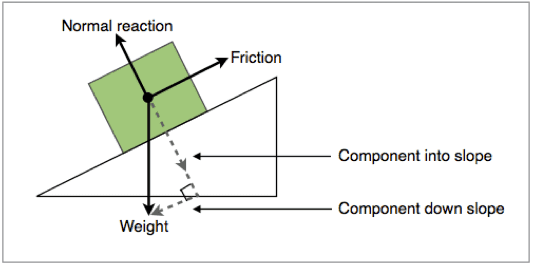Gravity acts downwards = mg
The component normal to the slope (into the slope) = mg*Cos(angle)
The component parallel to/down the slope = mg*Sin(angle)

The component normal or into the slope) is equivalent to the component in the direction of the conrod.

edit: If you have done the tip-to-tail method of adding vectors then perhaps you can see how the two orthogonal components add together to equal the weight vector. If they weren't orthogonal (at right angles) one component would itself have components in the direction of the other.

edit2: The very word "component" means "part of" so the component is smaller than the whole vector.

Last edited:
I have covered that before, but why is that the component in the direction of the conrod? the force here, mg is still the hypotenuse of the triangle right? and isn't the force down the conrod the hypotenuse?

CWatters
Homework Helper
Gold Member
I have covered that before, but why is that the component in the direction of the conrod? the force here, mg is still the hypotenuse of the triangle right?

Correct.

and isn't the force down the conrod the hypotenuse?

No. The hypotenuse is larger than the other sides of the triangle. How can a component be greater than the whole?

It's possible you are confusing the mechanical geometry (where the con rod does look like the hypotenuse) with a vector component diagram where it isn't.

Consider this diagram..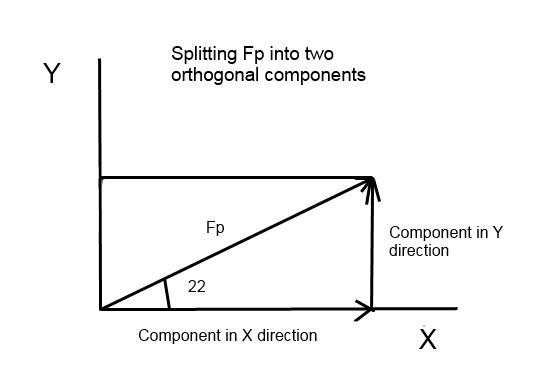I have marked a vector Fp and shown it's components in the X and Y directions. Does that make sense?

CWatters